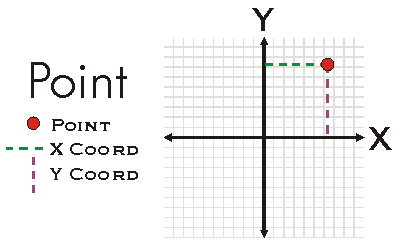ArcObjects Library Reference (Geometry)

# IPoint Interface

#### Product Availability

Available with ArcGIS Engine, ArcGIS Desktop, and ArcGIS Server.

#### Description

A Point is a zero-dimensional object that represents a specific (X, Y) location in a the two-dimensional XY-Plane.  A Point may also have Z, M, and ID attributes associated with it.  Existence of attributes does not alter the dimensionality of a Point nor does it alter geometric calculations performed on the Point.  Attributes are only considered for attribute calculations when the Point is ZAware, MAware, or PointIDAware.  Points may be constructed using PutCoords, individually setting the X and Y properties, or using the IConstructPoint interface.

#### Members

DescriptionCompare Compares X, Y, M, Z, ID of this point (in that order) with that of the other point. Returns -1 if this point's value is less, 1 if greater, and 0 otherwise. Useful for sorting a group of points.ConstrainAngle Projects this point to the point on the infinite line defined by anchor and angle (in radians). If allowOpposite is true, then the point can also snap to angle + pi radians.ConstrainDistance Projects this point to the perimeter of the circle defined by radius and anchor.Dimension The topological dimension of this geometry.Envelope Creates a copy of this geometry's envelope and returns it.GeometryType The type of this geometry.GeoNormalize Shifts longitudes, if need be, into a continuous range of 360 degrees.GeoNormalizeFromLongitude Normalizes longitudes into a continuous range containing the longitude. This method is obsolete.ID The Point ID attribute.IsEmpty Indicates whether this geometry contains any points.M The measure attribute.Project Projects this geometry into a new spatial reference.PutCoords Sets the X and Y coordinates.QueryCoords Returns the X and Y coordinates.QueryEnvelope Copies this geometry's envelope properties into the specified envelope.SetEmpty Removes all points from this geometry.SnapToSpatialReference Moves points of this geometry so that they can be represented in the precision of the geometry's associated spatial reference system.SpatialReference The spatial reference associated with this geometry.X The X coordinate.Y The Y coordinate.Z The Z attribute.

#### Inherited Interfaces

Interfaces Description
IGeometry Provides access to members that describe properties and behavior of all geometric objects.

#### CoClasses that implement IPoint

CoClasses and Classes Description
MapPoint (esriDataSourcesRaster) Implements interface IMapPoint (a point in map space).
Point A two dimensional point, optionally with measure, height, and ID attributes.

#### Remarks